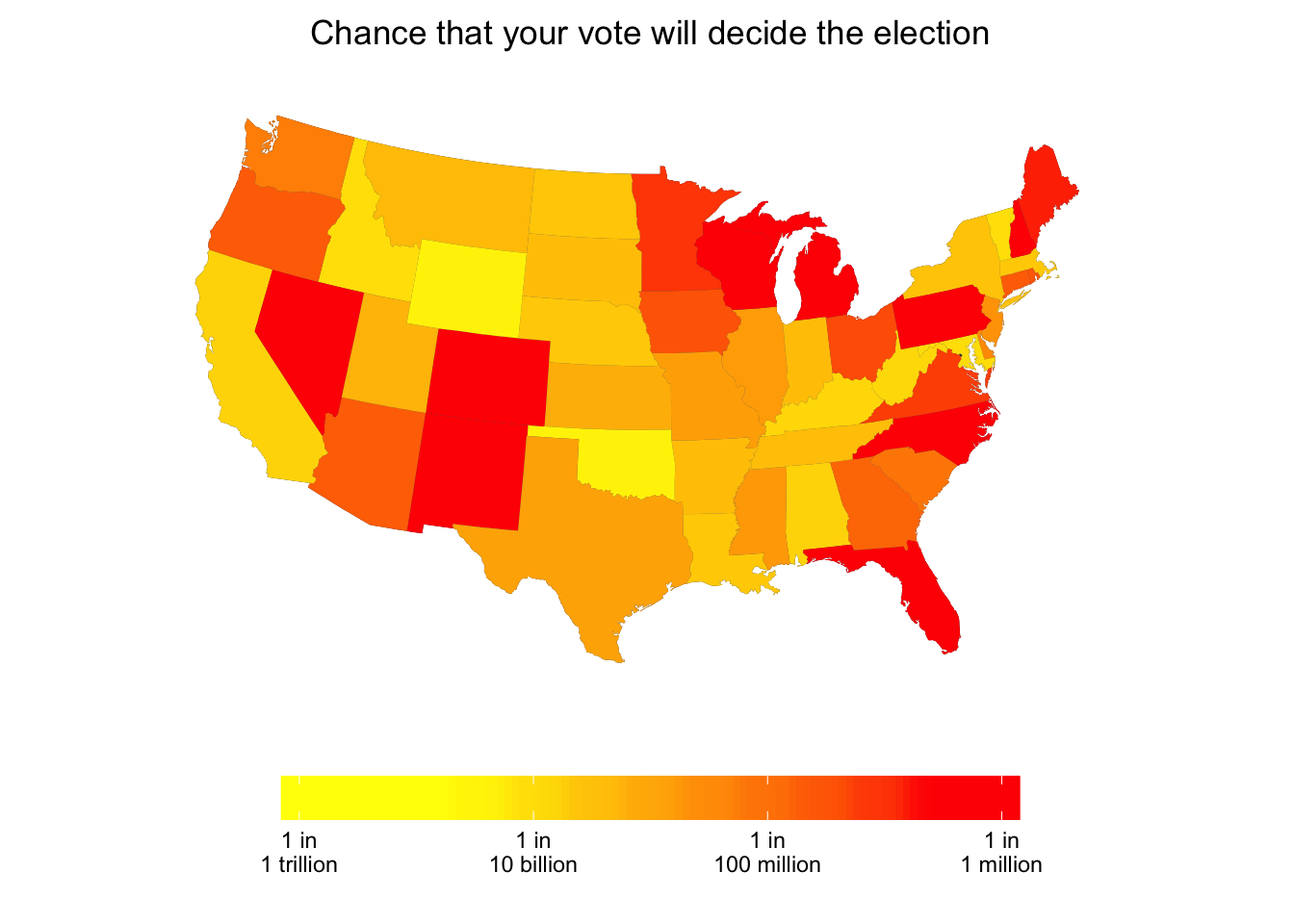Twitter: @pakremp

The probability that an individual voter would end up determining the outcome of the election is extremely low. But it varies dramatically across states. We use the posterior distribution of the state-by-state vote intentions forecast on election day to replicate the calculation detailed in Gelman, Silver and Edlin’s (2012) Economic Inquiry article, “What is the probability that your vote will make a difference?”.

The R code is available here.

The intuition behind this calculation is simple: your vote can decide the outcome of the election if and only if two conditions are met:

• The state in which you vote is critical to the outcome of the election – in other words, neither candidate has already reached a total of 270 electoral votes, without your state’s electoral votes. All things being equal, this situation is more likely if you live in a big state with a high number of electoral votes and if the electoral votes of other states are evenly split.

• The two leading candidates receive the exact same number of votes in your state. A highly improbable situation, of course, but which should be relatively more likely if the race is close and if turnout is low in your state.# How These Probabilities Were Calculated

To calculate this probabilities, we simply apply Bayes’s rule:

\begin{aligned} \textrm{Pr(your vote is decisive)} & = \textrm{Pr(your state's EV are critical} \cap \textrm{your state is tied)} \\ & = \textrm{Pr(your state's EV are critical)} \times \textrm{Pr(your state is tied | your state is critical)} \end{aligned}

• We calculate $$\textrm{Pr(your state's EV are critical)}$$ by calculating for each state $$i$$ the number of simulations from the posterior distribution of the state-by-state vote intentions forecast in which $$| \sum_{s \neq i} EV^{Clinton}[s] - EV^{Trump}[s]| < EV[i]$$, and adding half of the number of simulations in which $$| \sum_{s \neq i} EV^{Clinton}[s] - EV^{Trump}[s]| = EV[i]$$. ($$EV^{Clinton}[s]$$ and $$EV^{Trump}[s]$$ are the number of electoral votes already gained by Clinton or Trump in state $$s$$; $$EV[i]$$ is the number of electoral votes to be allocated by state $$i$$). We divide this number by the total number of simulations.

• The calculation of the conditional probability $$\textrm{Pr(your state is tied | your state is critical)}$$ is slightly more complicated. We take the set of simulations in which your state’s electoral votes are critical (simulations in which $$| \sum_{s \neq i} EV^{Clinton}[s] - EV^{Trump}[s] | \leq EV[i]$$) and calculate the mean $$M$$ and standard deviation $$S$$ of the Clinton net margin against Trump, in total number of votes. To do so, we use the 2012 election turnout numbers for state $$i$$, adjusted for adult population growth between 2011 and 2015 (according to state-by-state US Census data). The conditional probability of a tie is the density estimated at zero of a T distribution with location $$M$$ and scale $$S$$ and 4 degrees of freedom. Footnote 8 of Gelman, Silver and Edlin’s article explains why the T distribution with 4 degrees of freedom is preferable to the Normal distribution.

The following graph shows the conditional probability $$\textrm{Pr(your state is tied | your state's EV are critical)}$$ on the logarithmic x-axis, and the probability $$\textrm{Pr(your state's EV are critical)}$$ on the logarithmic y-axis.